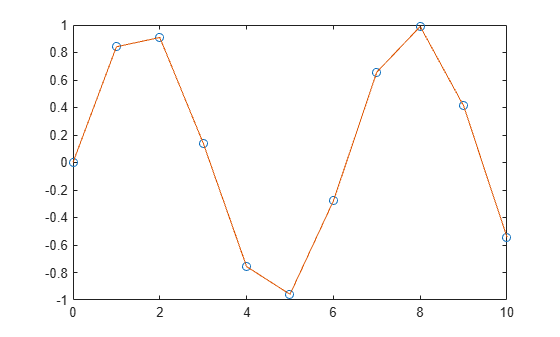# interp1q

Quick 1-D linear interpolation

## Compatibility

Note

`interp1q` is not recommended. Use `interp1` instead.

## Syntax

```yi = interp1q(x,Y,xi) ```

## Description

`yi = interp1q(x,Y,xi)` returns the value of the 1-D function `Y` at the points of column vector `xi` using linear interpolation. The vector `x` specifies the coordinates of the underlying interval. The length of output `yi` is equal to the length of `xi`.

For `interp1q` to work properly,

• `x` must be a monotonically increasing column vector.

• `Y` must be a column vector or matrix with `length(x)` rows.

• `xi` must be a column vector

`interp1q` returns NaN for any values of `xi` that lie outside the coordinates in `x`. If `Y` is a matrix, then the interpolation is performed for each column of `Y`, in which case `yi` is `length(xi)-by-size(Y,2)`.

## Examples

collapse all

Generate a coarse sine curve and interpolate over a finer abscissa.

```x = (0:10)'; y = sin(x); xi = (0:.25:10)'; yi = interp1q(x,y,xi); plot(x,y,'o',xi,yi)```